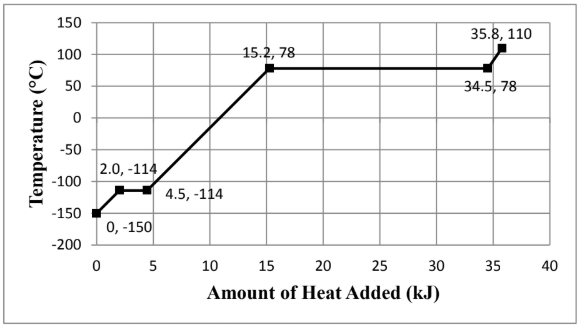# Problem: Consider the heating curve shown below for 22.86 grams of an unknown substance. You may assume constant pressure conditions. Note: the points are labeled as (amount of heat added, temperature). Calculate the enthalpy of fusion, ΔH fus, (in kJ/mol). Show all work and circle your final answer. The moles were identified to be 0.496 mol.

###### FREE Expert Solution

We are asked to the enthalpy of fusion, ΔH fus, (in kJ/mol) given the heating curve.

Phase changes occur on the horizontal parts of the curve since there are no temperature changes.

We go through the following steps to solve this problem:

Step 1. Calculate the heat to vaporize

Step 2. Calculate the molar heat of fusion

88% (15 ratings)###### Problem Details

Consider the heating curve shown below for 22.86 grams of an unknown substance. You may assume constant pressure conditions. Note: the points are labeled as (amount of heat added, temperature).Calculate the enthalpy of fusion, ΔH fus, (in kJ/mol). Show all work and circle your final answer. The moles were identified to be 0.496 mol.

Frequently Asked Questions

What scientific concept do you need to know in order to solve this problem?

Our tutors have indicated that to solve this problem you will need to apply the Heating and Cooling Curves concept. You can view video lessons to learn Heating and Cooling Curves. Or if you need more Heating and Cooling Curves practice, you can also practice Heating and Cooling Curves practice problems.